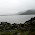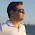Improve math skills of your kids - Learn step-by-step arithmetic from Math games

Math: Unknown - Step-by-step math calculation game for iOS.

Math: Unknown is much more than a math game. It is a step-by-step math calculation game which will teach users how to calculate in the correct order rather than just asking only the final calculated results.

The app consists of four basic arithmetic operations which are addition, subtraction, multiplication and division. In order to get started, users who are new to arithmetic can learn from animated calculation guides showing step-by-step procedures of solving each type of operation. It is also helpful for experienced users as a quick reference.

Generally, addition and subtraction may be difficult for users who just start learning math especially when questions require carrying or borrowing (also called regrouping). The app helps users to visualize the process of carrying and borrowing in the way it will be done on paper. Once users understand how these operations work, they are ready to learn multiplication and division.

For most students, division is considered as the most difficult arithmetic operation to solve. It is a common area of struggle since it requires prior knowledge of both multiplication and subtraction. To help users understand division, the app uses long division to teach all calculation procedures. Relevant multiplication table will be shown beside the question. Users will have to pick a number from the table which go into the dividend. Multiplication of selected number and divisor is automatically calculated, but the users have to do subtraction and drop down the next digit themselves. Learning whole calculation processes will make them master it in no time.

Math: Unknown is a helpful app for students who seriously want to improve arithmetic calculation skills.

Microsoft Excel VBA - Mohr's Circle for Plane Stress

The German engineer Otto Mohr (1835-1918) developed a useful pictorial or graphical interpretation of the equations for finding the principal stresses and the maximum shearing stress at a point in a stressed member. This method, commonly called Mohr's circle, involves the construction of a circle in such a manner that the coordinates of each point on the circle represent the normal and shearing stresses on one plane through the stressed point, and the angular position of the radius to the point gives the orientation of the plane.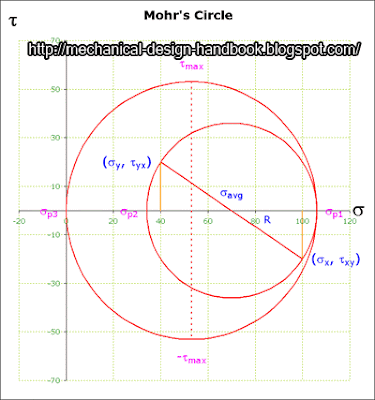Normal stresses are plotted as horizontal coordinates, with tensile stresses (positive) plotted to the right of the origin and compressive stresses (negative) plotted to the left. Shearing stresses are plotted as vertical coordinates, with those tending to produce a clockwise rotation of the stress element plotted above the s axis, and those tending to produce a counterclockwise rotation of the stress element plotted below the s axis.

The results obtained from Mohr's circle are identical with the equation derived from the free-body diagram. Consequently, Mohr's circle provides an extremely useful aid in the solution for, and visualization of, the stresses on various planes through a point in a stressed body in terms of the stresses on two mutually perpendicular planes through the point. Although Mohr's circle can be drawn to scale and used to obtain values of stresses and angles by direct measurements on the figure, it is probably more useful as a pictorial aid to the analyst who is performing analytical determinations of stresses and their directions at the point.dbf064 said…
How do i get it to show mohr's circle plotted in the negative x direction? The graph is not shown fully in the diagram.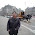Ake Blogger said…
Hi

You have to "Enable macros" when opening the excel file.

Please note that if you don't follow these steps, you may not be able to run the macro.

1) Tools > Macro > Security
2) Security Level tab > Medium. > OK
3) Close Microsoft Excel
4) Open the file again and you will see the pop-up
5) Select Enable Macros

Note: step 1 -3, you have to do only once, later you can skip them

I hope this helps.

Akestviolet said…
is it possible for you to include a square diagram with normal stress, shear stress and angels?

i get really confused when relating angle and rotation on square TO morh's circle's angles and rotation.

could you contact me if you can be bothered? slxia1@student.monash.edu.auRipu said…
HOW DO I GET TO ACCESS THE CODE WRITTEN. IT IS ASKING FOR A PASSWORD.gavin davis said…
Lovely program. few concerns, if anyone is still managing the comments. A mohr's stress circle should only be one circle with both the shear and normal stress axis to the same scale. I'm not quite sure what the second circle is for. The maximum shear stress for the values entered would be approx 129 Mpa. The angle for the maximum shear stress is correct but i'm not sure how this maximum shear stress value is being calculated or i'm mis-interpreting something.Justin Mayer said…
Is there a chance i could get a copy of the code?

j.mayer@my.und.edu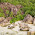Clairina Piink said…
Hi everybody
I'm a french student and I would like to have this program on excel for a project in mechanical engineering.

Please can someone send me this program.

Thanks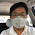To Ake Blogger,